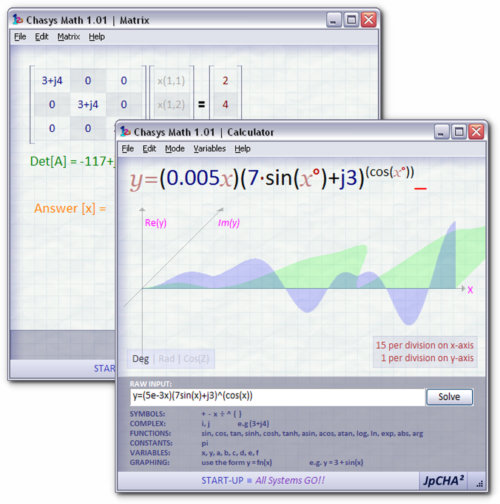# Solve equations, calculate complex numbers, with Chasys Math Tools

Chasys Math is a combination of free math utilities, the Chasys Math Calculator and the Chasys Math Matrix.

The calculator supports graph-plotting and complex numbers.The matrix equation solver can handle systems of equations as large as 32 by 32.